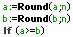KNOWLEDGE BASE
Tech Tip: Comparing Real Numbers to 'n' significant digits
PRODUCT: 4D | VERSION: | PLATFORM: Mac & Win
Published On: September 1, 2000

If you have a comparison statement such as:

and the 4D debugger shows the values of a = 14.53 and b = 14.53, then you might expect this expression to return True. However, even though the debugger shows the values to be equal we can only see two significant digits of these real numbers. If either of the values were calculated then with full precision 'a' might be 14.52999999756465. In this case, the expression would evaluate to False, despite the fact that the numbers appear equal when viewed in the debugger.

With full precision:

a = 14.52999999756465
b = 14.53

To solve this problem, and to make the expression return True, corresponding to the numbers displayed in the debugger, use the round function:This expression will evaluate TRUE. The round statements will round each value to n decimal places. n represents the number of decimals you would li ke to match.